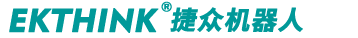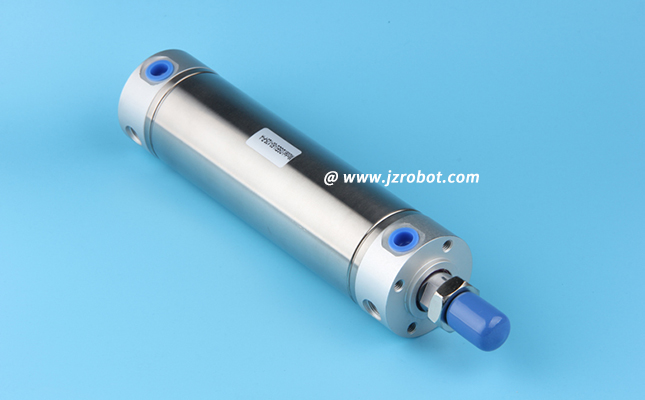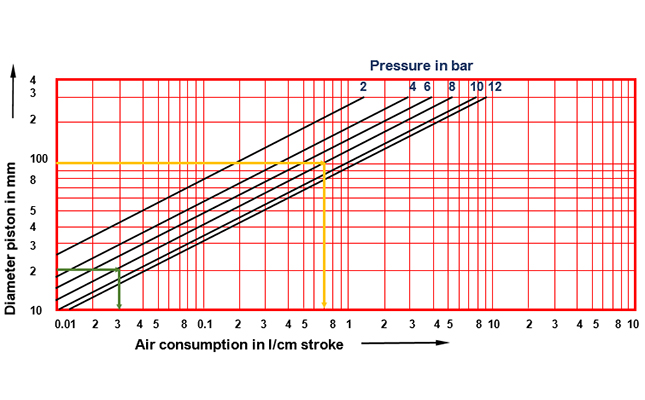## 气缸耗气量计算你会吗?不会的话,看这里

1、最大耗气量

qr=0.0462D^2*um(P+0.102)

PS：非官方经验公式：最大耗气量=缸径(cm计)平方再乘以系数10(此时使用压力为0.6MPa，最大速度为300mm/s)2、平均耗气量

qca=0.0157(D^2*L+d^2*ld)N(p+0.102)

qca：气缸的平均耗气量，L/min(ANR)；

N：气缸的工作频率，即每分钟内气缸的往复周数，一个往复为一周，周/min；

L：气缸的行程，cm；

d：换向阀与气缸之间的配管的内径，cm；

ld：配管的长度，cm。

qca=0.0157(D^2*L+d^2*ld)N(p+0.102)=0.0157*(10^2*10+1^2*6))*60*(0.6+0.102)=666.5251704L/min(ANR).